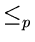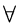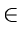Next:10.6 To Probe FurtherUp:10.5 NP-Complete ProblemsPrevious:10.5 NP-Complete Problems

## 10.5.1 NP-Hardness and NP-Completeness

A decision problem Y is said to be NP-hard if XNP. An NP-hard problem Y is said to be NP-complete if YNP. NPC is the standard notation for the class of all NP-complete problems.
• Informally, an NP-hard problem is a problem that is at least as hard as any problem in NP. If, further, the problem also belongs to NP, it would become NP-complete.
• It can be easily proved that if any NP-complete problem is in P, then NP = P. Similarly, if any problem in NP is not polynomial-time solvable, then no NP-complete problem will be polynomial-time solvable. Thus NP-completeness is at the crux of deciding whether or not NP = P.
• Using the above definition of NP-completeness to show that a given decision problem, say Y, is NP-complete will call for proving polynomial reducibility of each problem in NP to the problem Y. This is impractical since the class NP already has a large number of member problems and will continuously grow as researchers discover new members of NP.
• A more practical way of proving NP-completeness of a decision problem Y is to discover a problem XNPC such that XY. Since X is NP-complete andis a transitive relationship, the above would mean that ZNP. Furthermore if YNP, then Y is NP-complete.

• The above is the standard technique used for showing the NP-hardness or NP-completeness of a given decision problem. For example, all the decision problems given in Section 10.2 can be shown to be NP-complete by the above technique.

• An interesting question is: how was the first member of NPC found? Stephen Cook showed the NP-completeness of the problem 3-SAT by directly proving that X3-SATNP.
• If a problem is NP-complete, it does not mean that all hopes are lost. If the actual input sizes are small, an algorithm with, say, exponential running time may be acceptable. On the other hand, it may still be possible to obtain near-optimal solutions in polynomial-time. Such an algorithm that returns near-optimal solutions (in polynomial time) is called an approximation algorithm. Using Kruskal's algorithm to obtain a suboptimal solution to the TSP (see Chapter 8) is an example of this.Next:10.6 To Probe FurtherUp:10.5 NP-Complete ProblemsPrevious:10.5 NP-Complete Problems
eEL,CSA_Dept,IISc,Bangalore